Barrier potential of a P-N junction diode does not depend on

(a) Temperature                (b) Forward bias
(c) Doping density             (d) Diode design

Concept Questions :-

PN junction

Difficulty Level:

Which is the correct diagram of a half-wave rectifier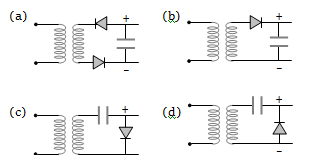Concept Questions :-

Rectifier

Difficulty Level:

The diode shown in the circuit is a silicon diode. The potential difference between the points A and B will be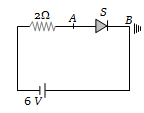(a) 6 V

(b) 0.6 V

(c) 0.7 V

(d) 0 V

Concept Questions :-

PN junction
High Yielding Test Series + Question Bank - NEET 2020

Difficulty Level:

Zener breakdown takes place if

(a) Doped impurity is low             (b) Doped impurity is high
(c) Less impurity in N-part           (d) Less impurity in P-type

Concept Questions :-

Applications of PN junction
High Yielding Test Series + Question Bank - NEET 2020

Difficulty Level:

The correct symbol for zener diode is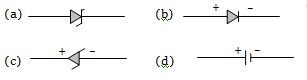Concept Questions :-

Applications of PN junction

Difficulty Level:

Which one of the following statements is not correct

(a) A diode does not obey Ohm's law

(b) A PN junction diode symbol shows an arrow identifying the direction of current (forward) flow

(c) An ideal diode is an open switch

(d) An ideal diode is an ideal one way conductor

Concept Questions :-

PN junction
High Yielding Test Series + Question Bank - NEET 2020

Difficulty Level:

A semiconductor X is made by doping a germanium crystal with arsenic (Z = 33). A second semiconductor Y is made by doping germanium with indium (Z = 49). The two are joined end to end and connected to a battery as shown. Which of the following statements is correct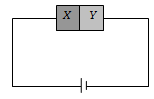(a) X is P-type, Y is N-type and the junction is forward biased

(b) X is N-type, Y is P-type and the junction is forward biased

(c) X is P-type, Y is N-type and the junction is reverse biased

(d) X is N-type, Y is P-type and the junction is reverse biased

Concept Questions :-

Types of semiconductors
High Yielding Test Series + Question Bank - NEET 2020

Difficulty Level:

Which is the wrong statement in following sentences?

A device in which P and N-type semiconductors are used is more useful then a vacuum type because

(a) Power is not necessary to heat the filament

(b) It is more stable

(c) Very less heat is produced in it

(d) Its efficiency is high due to a high voltage across the junction

Concept Questions :-

Applications of PN junction

Difficulty Level:

In the diagram, the input is across the terminals A and C and the output is across the terminals B and D, then the output is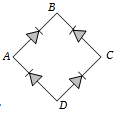(a) Zero
(b) Same as input
(c) Full wave rectifier
(d) Half wave rectifier

Concept Questions :-

Rectifier
High Yielding Test Series + Question Bank - NEET 2020

Difficulty Level:

The current through an ideal PN-junction shown in the following circuit diagram will be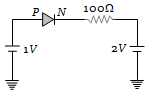(a) Zero
(b) 1 mA
(c) 10 mA
(d) 30 mA

Concept Questions :-

PN junction